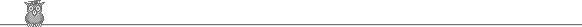### 35th IMO 1994 shortlistProblem C3

Peter has three accounts at a bank, each with an integral number of dollars. He is only allowed to transfer money from one account to another if the effect of the transfer is to double the amount of money in one of the accounts. Show that by a series of transfers he can always empty one account, but that he cannot always get all his money into one account.

Solution

The last part is trivial. Suppose all the money can be transferred to one account. Then the last move must be to double the money in that account. So Peter's total number of dollars must be even. If it is odd, then he cannot get all the money into one account.

Suppose the initial amount in each account is a ≤ b ≤ c. If a > 0 we show how to make transfers which end up with the smallest amount being reduced. That is evidently sufficient.

Call the account initially containing a, b, c the first, second, third account respectively. Write b = qa + r, with 0 ≤ r < a. Write q in binary: bk...b0. Now for i = 0, 1, ... , k make a transfer from the second account to the first if bi = 1 or a transfer from the third account to the first if bi = 0. In total, qa is transferred from the second account. Note also that at most 011...1 (k 1s) x a < bk0 ... 0 ≤ qa ≤ b ≤ c is transferred in total from the third account, so the transfers are possible. Since the second account is left with r < a, the effect of the transfers is to reduce the smallest amount, as claimed.